Calculators are NOT permitted.
Name *
-8 + 5 =
1 point
-10x(-7) =
1 point
The largest number in this group is
1 point
3.41 + 0.8
1 point
0.8 x 3 =
1 point
4 ÷ 0.1
1 point
1/6 + 2/3
1 point
4/3 ÷ 2
1 point
4² =
1 point
The square root of what number is between 4 and 5?
1 point
What percentage of the grid below is shaded?
1 point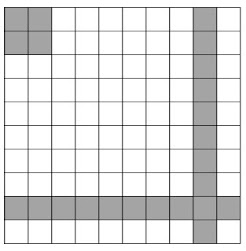1 point
-4 + 22 ÷ 2 =
1 point
2 + ( 8 - 3 ) x 5 - 2³ =
1 point
If a heart beats 144 times in 2 minutes, how many times will it beat in 5 minutes?
1 point
The ratio of girls to boys in a class is 3 to 5. If there are a total of 15 boys in the class, how many girls are there?
1 point
Find the total units in the perimeter of the shape below.
1 point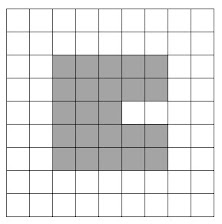Find the number of square units in the area of the shape below.
1 point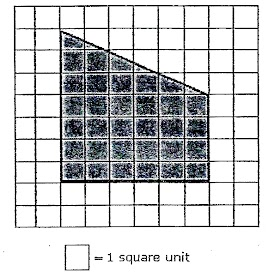On the grid below, which point is NOT on the grid?
1 point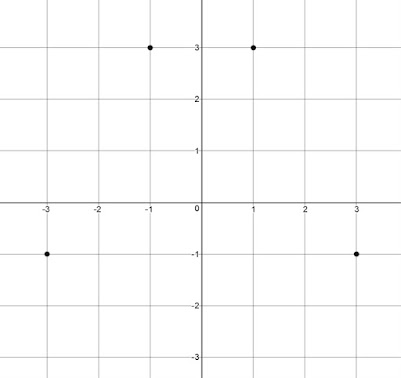From the graph below, determine which letter represents the drink with the most calories per mL?
1 point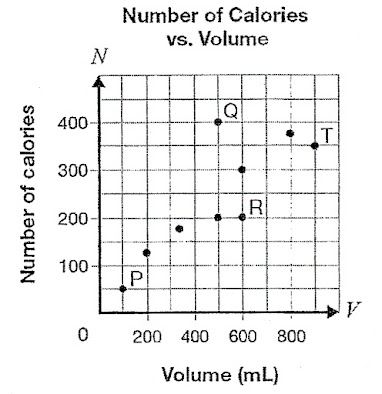If n = 8, 4n - 3 =
1 point
If 3n = 14, n is
1 point